Next: High Frequency Limit Up: Wave Propagation in Uniform Previous: Anomalous Dispersion and Resonant

# Wave Propagation in Conducting Media

In the limit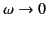, there is a significant difference in the response of a dielectric medium to an electromagnetic wave, depending on whether the lowest resonant frequency is zero or non-zero. For insulators, the lowest resonant frequency is different from zero. In this case, the low frequency refractive index is predominately real, and is also greater than unity. In a conducting medium, on the other hand, some fraction,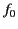, of the electrons are free,'' in the sense of having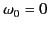. In this situation, the low frequency dielectric constant takes the form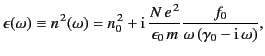(800)

where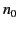is the contribution to the refractive index from all of the other resonances, and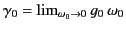. Consider the Ampère-Maxwell equation,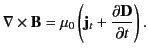(801)

Here,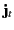is the true current: that is, the current carried by free, as opposed to bound, charges. Let us assume that the medium in question obeys Ohm's law,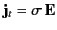, and has a normal'' dielectric constant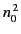. Here,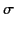is the conductivity. Assuming an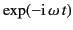time dependence of all field quantities, the previous equation yields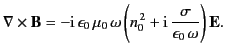(802)

Suppose, however, that we do not explicitly use Ohm's law but, instead, attribute all of the properties of the medium to the dielectric constant. In this case, the effective dielectric constant of the medium is equivalent to the term in round brackets on the right-hand side of the previous equation: that is,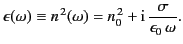(803)

A comparison of this term with Equation (801) yields the following expression for the conductivity,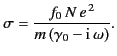(804)

Thus, at low frequencies, conductors possess predominately real conductivities (i.e., the current remains in phase with the electric field). However, at higher frequencies, the conductivity becomes complex. At such frequencies, there is little meaningful distinction between a conductor and an insulator, because the conductivity'' contribution to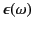appears as a resonant amplitude, just like the other contributions. For a good conductor, such as copper, the conductivity remains predominately real for all frequencies up to, and including, those in the microwave region of the electromagnetic spectrum.

The conventional way in which to represent the complex refractive index of a conducting medium (in the low frequency limit) is to write it in terms of a real normal'' dielectric constant,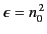, and a real conductivity,. Thus, from Equation (804),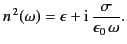(805)

For a poor conductor (i.e.,), we find that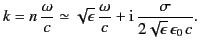(806)

In this limit,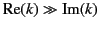, and the attenuation of the wave, which is governed by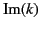[see Equation (776)], is independent of the frequency. Thus, for a poor conductor, the wave acts like a wave propagating through a conventional dielectric of dielectric constant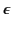, except that it attenuates gradually over a distance of very many wavelengths. For a good conductor (i.e.,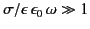), we obtain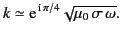(807)

It follows from Equation (772) that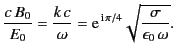(808)

Thus, the phase of the magnetic field lags that of the electric field by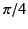radians. Moreover, the magnitude of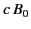is much larger than that of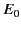(because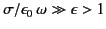). It follows that the wave energy is almost entirely magnetic in nature. Clearly, an electromagnetic wave propagating through a good conductor has markedly different properties to a wave propagating through a conventional dielectric. For a wave propagating in the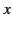-direction, the amplitudes of the electric and magnetic fields attenuate like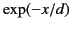, where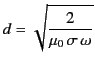(809)

is termed the skin depth. It is apparent that an electromagnetic wave incident on a conducting medium will not penetrate more than a few skin depths into that medium.Next: High Frequency Limit Up: Wave Propagation in Uniform Previous: Anomalous Dispersion and Resonant
Richard Fitzpatrick 2014-06-27